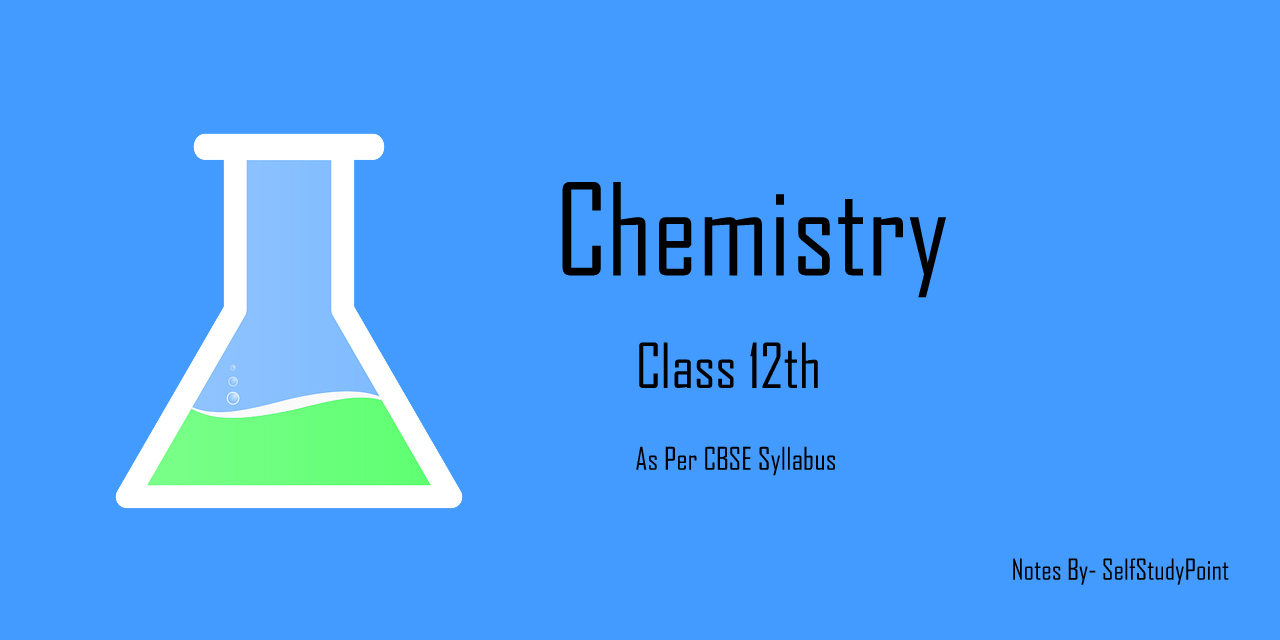# Expressing Concentration of Solutions

The composition of a solution is defined in terms of concentration. There are several ways to define the concentration of the solution as follows:

## Mass percentage (w/w):

The mass percentage of a component of a solution is defined as: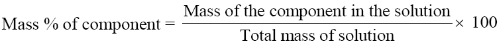## Volume percentage (v/v):

The volume percentage is defined as: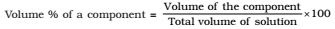## Mass by volume percentage (w/v):

Another unit which is commonly used in medicine and pharmacy is mass by volume percentage. It is the mass of solute dissolved in 100 mL of the solution.## Part per million (ppm):

When a solute is present in trace quantities, it is convenient to express concentration in parts per million (ppm).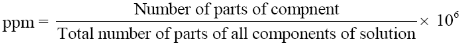## Mole fraction:

A commonly used symbol for mole fraction is x and subscript used on the right-hand side of x denotes the component.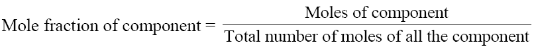Molarity:

Molarity (M) is defined as a number of moles of solute dissolved in one litre (or one cubic decimeter) of the solution,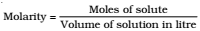## Molality:

Molality (m) is defined as the number of moles of the solute per kilogram (kg) of the solvent and is expressed as: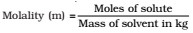Click on a star to rate it!

Average rating 5 / 5. Vote count: 2

No votes so far! Be the first to rate this post.

As you found this post useful...